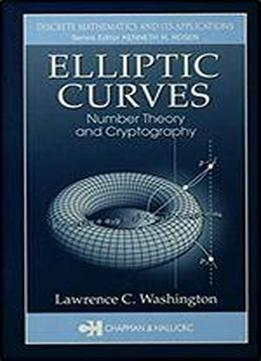# Elliptic Curves: Number Theory And Cryptography (discrete Mathematics And Its Applications) by Lawrence C. Washington / 2003 / English / EPUB

Elliptic curves have played an increasingly important role in number theory and related fields over the last several decades, most notably in areas such as cryptography, factorization, and the proof of Fermat's Last Theorem. However, most books on the subject assume a rather high level of mathematical sophistication, and few are truly accessible to senior undergraduate or beginning graduate students. Assuming only a modest background in elementary number theory, groups, and fields, Elliptic Curves: Number Theory and Cryptography introduces both the cryptographic and number theoretic sides of elliptic curves, interweaving the theory of elliptic curves with their applications. The author introduces elliptic curves over finite fields early in the treatment, leading readers directly to the intriguing cryptographic applications, but the book is structured so that readers can explore the number theoretic aspects independently if desired. By side-stepping algebraic geometry in favor an approach based on basic formulas, this book clearly demonstrates how elliptic curves are used and opens the doors to higher-level studies. Elliptic Curves offers a solid introduction to the mathematics and applications of elliptic curves that well prepares its readers to tackle more advanced problems in cryptography and number theory.

views: 530